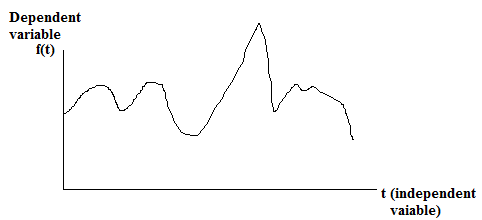### Signal and Systems tutorials – lecture 1.1

The following series of lectures about the signals and systems gives the brief idea about signals and their analysis for beginners. These lectures also help to understand the difference between time domain, frequency domain and spacial domain. A clear idea will be presented about importance of Fourier series and Fourier transforms to analyze the signals. Further, design of stable systems for signal processing using z-transforms. Finally a small real time project called digital sthescope will be presented to analyze the heart beat signals along with other noise signals.
i.     Introduction to signals

What is a signal?
A signal is any physical phenomenon (like gesture, action, electrical impulse, sound, image and video) through which one can convey information to other. In other words, signals are functions of one or more independent variables typically carry some type of information. The graphical representation of signal is shown in the figure 1.1figure 1.1 A one dimension continous time signal representation

Types of signals:
Generally, Signals are classified into two types
1.    Continuous signals:
These signals are functions of continuous independent variable or real number. Again these continuous signals are categorized into two.
a.       One dimension continuous signals:
One dimension continuous signals are the function of continuous single independent variable. For, example a speech signal is a continuous one-dimension signal, where time (t) is the independent variable. Hence speech signal is referred as continuous time one- dimensional signal. Human speech signal is shown in figure 1.2figure 1.2 human speech signal representation

b.      Multi-dimension continuous signals:
Multi-dimension continuous signals are the function of multiple continuous independent variables. For, example image brightness is a function that varies horizontally and vertically.
brightness(horiz,verti);
2.    Discrete signals:
These signals are functions of integer number or discrete independent variable. The discrete signals are also categorized into two.
a.       One dimension discrete signals:
One dimension discrete signals are the function of discrete single independent variable. The representation of discrete time signal is shown below figure 1.3.figure 1.3 one dimension discrete signal representation

b.      Multi-dimension discrete signals:
Multi-dimension discrete signals are the function of multiple discrete independent variables. For example, the representation of spatial antenna array is a two dimension discrete signal x[n,m].

#### 1 comment :

1.great post. thanks for sharing this information
KineMaster for pc
Free fire for pc
vhyj

Thanks for visiting NPEDUCATIONS. We will contact you soon

#### 1 comments :

jaastark said...

great post. thanks for sharing this information
KineMaster for pc
Free fire for pc
vhyj